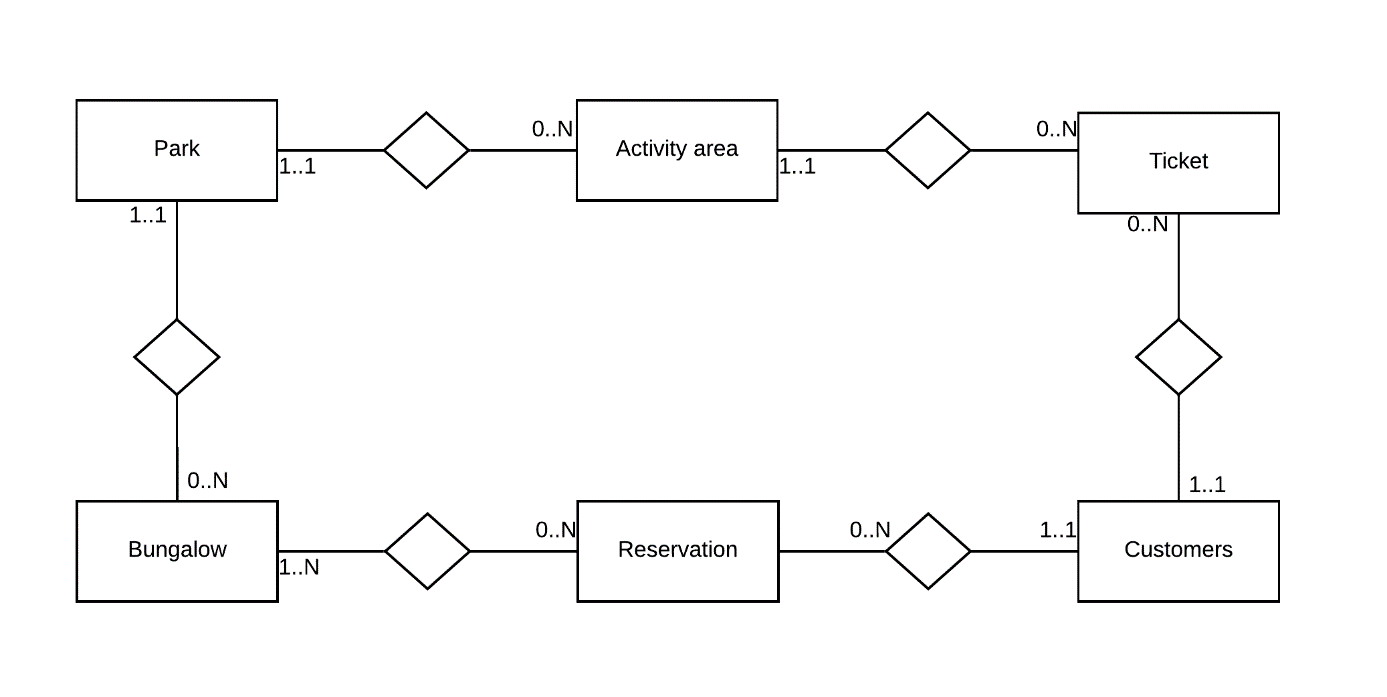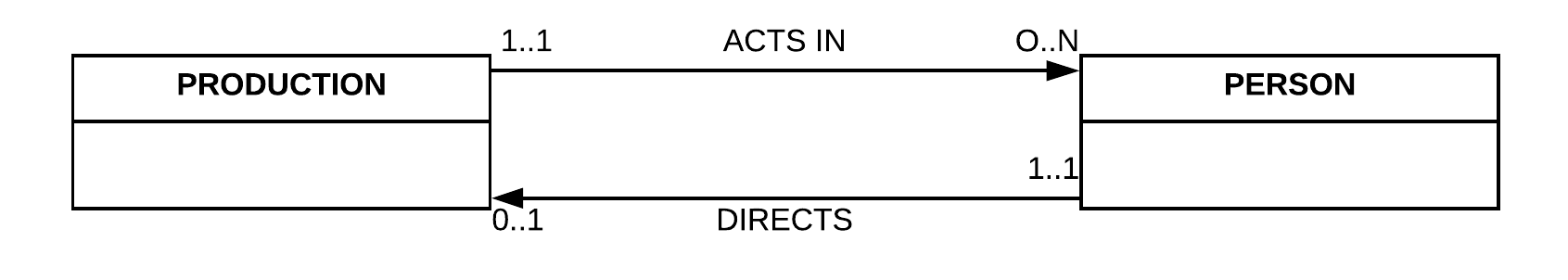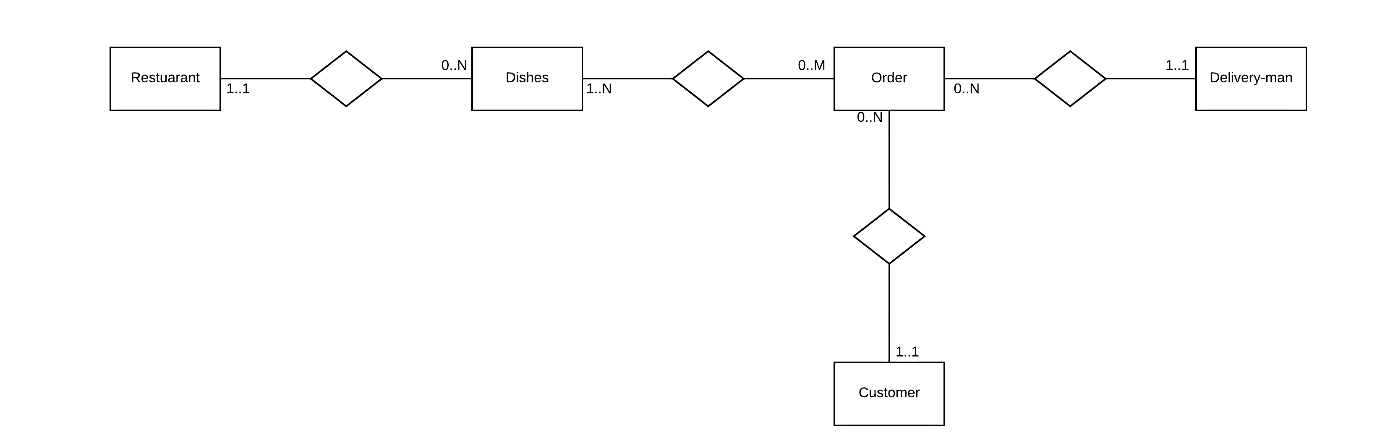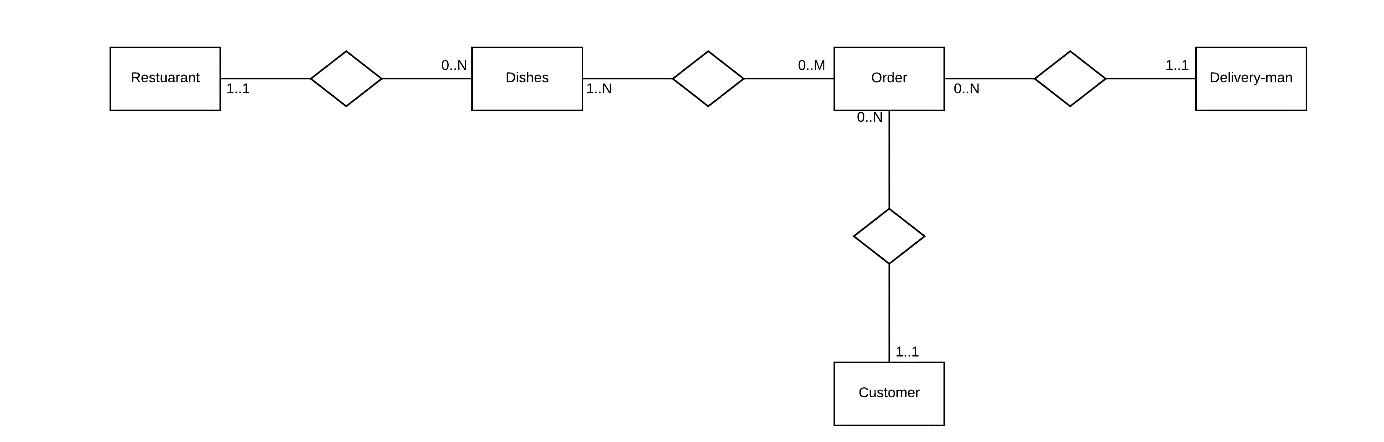MC If a hierarchical model contains N:M relationship types that have been integrated by repeating the child nodes where necessary, what are the dangers of updating the database? Slower retrieval of data incorrect Creating data inconsistency correct Creating unnecessary records incorrect All of the above incorrect MC How can the CODASYL framework correctly model a family tree, taking into account that every child has to have (at least) two parents? Use a dummy record type 'is parent of' between record types 'parent' and 'child'. correct We cannot model this constraint with the CODASYL framework. incorrect Use a set type 'is parent of' between record types 'parent' and 'child'. incorrect List the parents as a vector in the data item set of every child. incorrect MCWhich of the following statements on turning the above EER model into a CODASYL model is correct? We have to introduce 6 record types. incorrect We cannot enforce that a reservation needs at least one customer. correct We have to introduce 8 record types. incorrect All of the above statements are correct. incorrect MC In CODASYL, a multivalued composite attribute type can be represented as a: Data item incorrect Vector incorrect Repeated group correct Record type incorrect MCHow many of these above cardinalitites can be enforced in the CODASYL model? 5 incorrect 6 incorrect 7 correct 8 incorrect MCWhich of the following statements on turning the above EER model into a hierarchical model is correct ? All cardinalities can be enforced. incorrect We have to introduce a virtual child record type to model the relationship between 'Dishes' and 'Order'. correct We have to introduce a virtual child record type to model the relationship between 'Restaurant' and 'Dishes'. incorrect We cannot enforce that an order has exactly one customer. incorrect MC How can the CODASYL framework model a person's e-mail address (assuming a person can have more than one e-mail address)? Use a repeated group. incorrect Introduce a virtual child for each mail address. incorrect We cannot model this in the CODASYL framework. incorrect Use a vector. correct MCIf we would turn the above EER model into a hierarchical model, how many record types would we have to introduce? 7 incorrect 6 correct 5 incorrect 8 incorrect MC How can the CODASYL framework model a person's e-mail address (assuming a person can have more than one e-mail address)? Use a vector. correct We cannot model this in the CODASYL framework. incorrect Use a repeated group. incorrect Introduce a virtual child for each mail address. incorrect MCWhich of the following statements on turning the above EER model into a CODASYL model is correct? We have to introduce 6 record types. incorrect We cannot enforce that a reservation needs at least one customer. correct We have to introduce 8 record types. incorrect All of the above statements are correct. incorrect